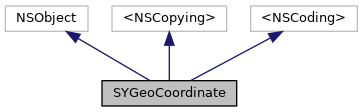SYGeoCoordinate Class Reference

Represents WGS84 coordinates with double precision. More...

`#import <SYPositioning.h>`

Inheritance diagram for SYGeoCoordinate:[legend]
Collaboration diagram for SYGeoCoordinate:[legend]

## Instance Methods

(nonnull instancetype) - initWithLatitude:longitude:
Initializes a SYGeoCoordinates instance with specified latitude and longitude values. More...

(nonnull instancetype) - initWithLatitude:longitude:altitude:
Initializes a SYGeoCoordinates instance with specified values for the initial latitude, longitude and altitude. More...

(BOOL) - isValid
Validates latitude and longitude values. More...

(SYDistance) - distanceToGeoCoordinate:
Calculates air distance to specified SYGeoCoordinate. More...

## Properties

double latitude
The latitude, as measured in degrees, representing north-south coordinate (y-axis on a map). The latitude must be in the range [-90, 90]. Values outside this range will be clamped. More...

double longitude
The longitude, as measured in degrees, representing east-west coordinate (x-axis on a map). The longitude must be in the range [-180, 180]. More...

double altitude
The altitude of the SYGeoCoordinates measured in meters above sea-level. Geocoordinates with positive altitudes represent points above the plane of the map, while negative altitudes are below the plane of the map. Map objects with these coordinates will be displayed accordingly. Valid values are in the range [-10000.0, 10000.0]. More...

## Detailed Description

Represents WGS84 coordinates with double precision.

## ◆ initWithLatitude:longitude:

 - (nonnull instancetype) initWithLatitude: (double) latitude longitude: (double) longitude

Initializes a SYGeoCoordinates instance with specified latitude and longitude values.

## ◆ initWithLatitude:longitude:altitude:

 - (nonnull instancetype) initWithLatitude: (double) latitude longitude: (double) longitude altitude: (double) altitude

Initializes a SYGeoCoordinates instance with specified values for the initial latitude, longitude and altitude.

## ◆ isValid

 - (BOOL) isValid

Validates latitude and longitude values.

## ◆ distanceToGeoCoordinate:

 - (SYDistance) distanceToGeoCoordinate: (nonnull SYGeoCoordinate *) coordinate

Calculates air distance to specified SYGeoCoordinate.

## ◆ latitude

 - (double) latitude

The latitude, as measured in degrees, representing north-south coordinate (y-axis on a map). The latitude must be in the range [-90, 90]. Values outside this range will be clamped.

## ◆ longitude

 - (double) longitude